SPECIFICATION PROBLEMS: Part 1

Omission of a Relevant Variable

Suppose that the true model is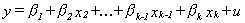but through some error in judgement we estimate the parameters of the model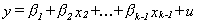That is we incorrectly omit the kth independent variable. What are the consequences of having omitted the kth variable? Define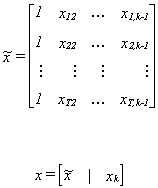The OLS estimate from the incorrectly specified model is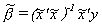To check for bias we will substitute in for y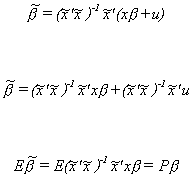Let us take a closer look at P. Begin with the product of the design matrix with the last column omitted and the correct design matrix.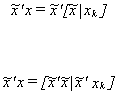Substitute back into the estimator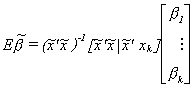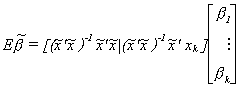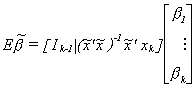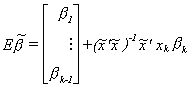Now consider the second term. Except for bk, it looks like an OLS estimator resulting from regressing xk on the remaining independent variables. That is,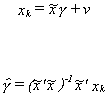So the bias of the least squares estimator for the omitted variables model is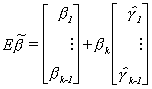The LS estimator is biased except when either

1. bk = 0
or
2.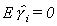for all i. If this is the case then xk is linearly independent of all the other columns of x.
or
3. Suppose xk is uncorrelated with the other columns of x, then the slopes are unbiased but the intercept is biased unless the mean of xk is also zero.

Inclusion of an Irrelevant Variable
No problem, Mon. If an irrelevant variable is included, then in expectation it takes the value of zero and has no impact on the correctly included variables. Nor, in expectation, does it change the efficiency of least squares. However, we don't live in a perfect long run world. Consequently, since including more variables necessarily reduces the residual sum of squares, the estimate of the coefficient variance will be smaller.   In practice we may find ourselves rejecting too many null hypotheses.

 Specification ToC Functional Form Dummies and Splines Non-nested Hypotheses Home 615 Syllabus 616 Syllabus Lecture Notes ToC